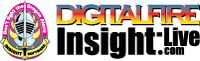•The secret to cool bodies and glazes is a lot of testing.
•The secret to know what to test is material and chemistry knowledge.
•The secret to learning from testing is documentation.
•The place to test, do the chemistry and document is an account at https://insight-live.com
•The place to get the knowledge is https://digitalfire.com

Sign-up at https://insight-live.com today.

# Christy Plastic Fireclay

 Oxide Analysis Formula CaO 0.32% 0.015 MgO 0.71% 0.047 K2O 1.45% 0.041 Na2O 0.16% 0.007 P2O5 0.08% 0.002 TiO2 2.06% 0.069 Al2O3 38.22% 1.000 SiO2 55.40% 2.460 Fe2O3 1.56% 0.026 SO3 0.03 Oxide Weight 266.77 Formula Weight 266.85

## XML for Import into INSIGHT

<?xml version="1.0" encoding="UTF-8"?> <material name="Christy Plastic Fireclay" descrip="" searchkey="" loi="0.03" casnumber="70694-09-6"> <oxides> <oxide symbol="CaO" name="Calcium Oxide, Calcia" status="" percent="0.320" tolerance=""/> <oxide symbol="MgO" name="Magnesium Oxide, Magnesia" status="" percent="0.710" tolerance=""/> <oxide symbol="K2O" name="Potassium Oxide" status="" percent="1.450" tolerance=""/> <oxide symbol="Na2O" name="Sodium Oxide, Soda" status="" percent="0.160" tolerance=""/> <oxide symbol="P2O5" name="Phosphorus Pentoxide" status="" percent="0.080" tolerance=""/> <oxide symbol="TiO2" name="Titanium Dioxide, Titania" status="" percent="2.060" tolerance=""/> <oxide symbol="Al2O3" name="Aluminum Oxide, Alumina" status="U" percent="38.220" tolerance=""/> <oxide symbol="SiO2" name="Silicon Dioxide, Silica" status="" percent="55.400" tolerance=""/> <oxide symbol="Fe2O3" name="Iron Oxide, Ferric Oxide" status="" percent="1.560" tolerance=""/> </oxides> <volatiles> <volatile symbol="SO3" name="Sulfur Trioxide" percent="0.030" tolerance=""/> </volatiles> </material>